# Mean State

Period Mean (original grids) [K]
Model Period Mean (intersection) [K]
Model Period Mean (complement) [K]
Benchmark Period Mean (intersection) [K]
Benchmark Period Mean (complement) [K]
Bias [K]
RMSE [K]
Phase Shift [months]
Bias Score 
RMSE Score 
Seasonal Cycle Score 
Spatial Distribution Score 
Interannual Variability Score 
Overall Score 
Benchmark [-] 299.
CLM4 [-] 298. 298. 300. 298. 300. -0.207 0.914 0.514 0.54 0.68 0.95 0.99 0.91 0.79
CLM4.5 [-] 298. 298. 300. 298. 300. -0.00920 0.830 0.455 0.58 0.71 0.96 1.0 0.93 0.81
CLM5 [-] 299. 299. 300. 298. 300. 0.285 0.690 0.366 0.67 0.78 0.97 1.0 0.96 0.86
Period Mean (original grids) [K]
Model Period Mean (intersection) [K]
Model Period Mean (complement) [K]
Benchmark Period Mean (intersection) [K]
Benchmark Period Mean (complement) [K]
Bias [K]
RMSE [K]
Phase Shift [months]
Bias Score 
RMSE Score 
Seasonal Cycle Score 
Spatial Distribution Score 
Interannual Variability Score 
Overall Score 
Benchmark [-] 300.
CLM4 [-] 300. 300. 300. 300. 300. 0.0637 0.861 0.221 0.79 0.78 0.98 0.99 0.94 0.88
CLM4.5 [-] 300. 300. 300. 300. 300. 0.276 0.853 0.188 0.76 0.82 0.99 0.99 0.96 0.89
CLM5 [-] 301. 301. 301. 300. 300. 0.473 0.802 0.168 0.75 0.86 0.99 0.99 0.96 0.90
Period Mean (original grids) [K]
Model Period Mean (intersection) [K]
Model Period Mean (complement) [K]
Benchmark Period Mean (intersection) [K]
Benchmark Period Mean (complement) [K]
Bias [K]
RMSE [K]
Phase Shift [months]
Bias Score 
RMSE Score 
Seasonal Cycle Score 
Spatial Distribution Score 
Interannual Variability Score 
Overall Score 
Benchmark [-] 295.
CLM4 [-] 295. 295. 296. 295. 297. 0.186 0.864 0.332 0.74 0.80 0.96 1.0 0.94 0.87
CLM4.5 [-] 295. 295. 296. 295. 297. 0.367 0.842 0.267 0.73 0.84 0.97 1.0 0.95 0.89
CLM5 [-] 296. 296. 296. 295. 297. 0.505 0.854 0.273 0.71 0.87 0.97 1.0 0.96 0.89
Period Mean (original grids) [K]
Model Period Mean (intersection) [K]
Model Period Mean (complement) [K]
Benchmark Period Mean (intersection) [K]
Benchmark Period Mean (complement) [K]
Bias [K]
RMSE [K]
Phase Shift [months]
Bias Score 
RMSE Score 
Seasonal Cycle Score 
Spatial Distribution Score 
Interannual Variability Score 
Overall Score 
Benchmark [-] 262.
CLM4 [-] 261. 261. 266. 262. 266. -0.321 1.44 0.0136 0.93 0.94 1.0 1.0 0.93 0.96
CLM4.5 [-] 261. 261. 266. 262. 266. -0.204 1.36 0.0125 0.94 0.94 1.0 1.0 0.93 0.96
CLM5 [-] 262. 261. 267. 262. 266. 0.258 1.13 0.0161 0.94 0.96 1.0 1.0 0.94 0.97
Period Mean (original grids) [K]
Model Period Mean (intersection) [K]
Model Period Mean (complement) [K]
Benchmark Period Mean (intersection) [K]
Benchmark Period Mean (complement) [K]
Bias [K]
RMSE [K]
Phase Shift [months]
Bias Score 
RMSE Score 
Seasonal Cycle Score 
Spatial Distribution Score 
Interannual Variability Score 
Overall Score 
Benchmark [-] 299.
CLM4 [-] 299. 299. 299. 299. 299. -0.368 0.940 0.699 0.31 0.59 0.90 0.94 0.91 0.70
CLM4.5 [-] 299. 299. 299. 299. 299. -0.197 0.851 0.662 0.36 0.62 0.90 0.93 0.91 0.72
CLM5 [-] 299. 299. 300. 299. 299. 0.226 0.750 0.486 0.42 0.72 0.93 0.96 0.91 0.78
Period Mean (original grids) [K]
Model Period Mean (intersection) [K]
Model Period Mean (complement) [K]
Benchmark Period Mean (intersection) [K]
Benchmark Period Mean (complement) [K]
Bias [K]
RMSE [K]
Phase Shift [months]
Bias Score 
RMSE Score 
Seasonal Cycle Score 
Spatial Distribution Score 
Interannual Variability Score 
Overall Score 
Benchmark [-] 271.
CLM4 [-] 270. 270. 274. 271. 274. -0.783 1.25 0.00895 0.94 0.94 1.0 1.0 0.97 0.97
CLM4.5 [-] 271. 270. 274. 271. 274. -0.735 1.22 0.00895 0.94 0.95 1.0 1.0 0.97 0.97
CLM5 [-] 271. 271. 275. 271. 274. -0.0249 0.647 0.00842 0.97 0.97 1.0 1.0 0.98 0.98
Period Mean (original grids) [K]
Model Period Mean (intersection) [K]
Model Period Mean (complement) [K]
Benchmark Period Mean (intersection) [K]
Benchmark Period Mean (complement) [K]
Bias [K]
RMSE [K]
Phase Shift [months]
Bias Score 
RMSE Score 
Seasonal Cycle Score 
Spatial Distribution Score 
Interannual Variability Score 
Overall Score 
Benchmark [-] 280.
CLM4 [-] 280. 280. 288. 280. 285. -0.256 1.33 0.0234 0.89 0.93 1.0 1.0 0.95 0.95
CLM4.5 [-] 280. 280. 288. 280. 285. -0.186 1.30 0.0230 0.89 0.93 1.0 1.0 0.95 0.95
CLM5 [-] 281. 280. 288. 280. 285. 0.249 1.09 0.0222 0.90 0.96 1.0 1.0 0.96 0.96
Period Mean (original grids) [K]
Model Period Mean (intersection) [K]
Model Period Mean (complement) [K]
Benchmark Period Mean (intersection) [K]
Benchmark Period Mean (complement) [K]
Bias [K]
RMSE [K]
Phase Shift [months]
Bias Score 
RMSE Score 
Seasonal Cycle Score 
Spatial Distribution Score 
Interannual Variability Score 
Overall Score 
Benchmark [-] 297.
CLM4 [-] 296. 296. 300. 297. 300. -0.473 1.33 0.200 0.75 0.82 0.98 1.0 0.94 0.88
CLM4.5 [-] 297. 297. 300. 297. 300. -0.254 1.23 0.191 0.76 0.84 0.98 1.0 0.94 0.89
CLM5 [-] 297. 297. 300. 297. 300. 0.110 1.08 0.181 0.77 0.88 0.98 1.0 0.95 0.91
Period Mean (original grids) [K]
Model Period Mean (intersection) [K]
Model Period Mean (complement) [K]
Benchmark Period Mean (intersection) [K]
Benchmark Period Mean (complement) [K]
Bias [K]
RMSE [K]
Phase Shift [months]
Bias Score 
RMSE Score 
Seasonal Cycle Score 
Spatial Distribution Score 
Interannual Variability Score 
Overall Score 
Benchmark [-] 264.
CLM4 [-] 263. 263. 266. 264. 264. -0.568 1.27 0.0103 0.95 0.94 1.0 1.0 0.95 0.96
CLM4.5 [-] 263. 263. 266. 264. 264. -0.485 1.14 0.0109 0.95 0.95 1.0 1.0 0.95 0.97
CLM5 [-] 264. 264. 267. 264. 264. 0.0517 0.717 0.0135 0.97 0.97 1.0 1.0 0.96 0.98
Period Mean (original grids) [K]
Model Period Mean (intersection) [K]
Model Period Mean (complement) [K]
Benchmark Period Mean (intersection) [K]
Benchmark Period Mean (complement) [K]
Bias [K]
RMSE [K]
Phase Shift [months]
Bias Score 
RMSE Score 
Seasonal Cycle Score 
Spatial Distribution Score 
Interannual Variability Score 
Overall Score 
Benchmark [-] 295.
CLM4 [-] 295. 295. 293. 295. 294. 0.233 0.671 0.0903 0.90 0.91 0.99 1.0 0.97 0.95
CLM4.5 [-] 295. 295. 293. 295. 294. 0.234 0.671 0.0898 0.90 0.91 0.99 1.0 0.97 0.95
CLM5 [-] 295. 295. 294. 295. 294. 0.479 0.699 0.0807 0.88 0.93 0.99 1.0 0.97 0.95
Period Mean (original grids) [K]
Model Period Mean (intersection) [K]
Model Period Mean (complement) [K]
Benchmark Period Mean (intersection) [K]
Benchmark Period Mean (complement) [K]
Bias [K]
RMSE [K]
Phase Shift [months]
Bias Score 
RMSE Score 
Seasonal Cycle Score 
Spatial Distribution Score 
Interannual Variability Score 
Overall Score 
Benchmark [-] 282.
CLM4 [-] 281. 281. 284. 282. 286. -0.398 1.19 0.130 0.88 0.90 0.99 1.0 0.95 0.94
CLM4.5 [-] 281. 281. 284. 282. 286. -0.410 1.16 0.127 0.88 0.90 0.99 1.0 0.96 0.94
CLM5 [-] 282. 282. 284. 282. 286. 0.117 0.866 0.145 0.90 0.94 0.99 1.0 0.97 0.96
Period Mean (original grids) [K]
Model Period Mean (intersection) [K]
Model Period Mean (complement) [K]
Benchmark Period Mean (intersection) [K]
Benchmark Period Mean (complement) [K]
Bias [K]
RMSE [K]
Phase Shift [months]
Bias Score 
RMSE Score 
Seasonal Cycle Score 
Spatial Distribution Score 
Interannual Variability Score 
Overall Score 
Benchmark [-] 287.
CLM4 [-] 282. 286. 248. 287. 292. -0.253 1.12 0.171 0.81 0.86 0.98 1.0 0.95 0.91
CLM4.5 [-] 282. 286. 248. 287. 292. -0.166 1.08 0.158 0.82 0.87 0.98 1.0 0.95 0.92
CLM5 [-] 283. 287. 249. 287. 292. 0.227 0.898 0.137 0.83 0.91 0.99 1.0 0.96 0.93
Period Mean (original grids) [K]
Model Period Mean (intersection) [K]
Model Period Mean (complement) [K]
Benchmark Period Mean (intersection) [K]
Benchmark Period Mean (complement) [K]
Bias [K]
RMSE [K]
Phase Shift [months]
Bias Score 
RMSE Score 
Seasonal Cycle Score 
Spatial Distribution Score 
Interannual Variability Score 
Overall Score 
Benchmark [-] 296.
CLM4 [-] 296. 296. 296. 296. 297. -0.135 0.977 0.177 0.89 0.92 0.99 1.0 0.96 0.94
CLM4.5 [-] 296. 296. 296. 296. 297. -0.0491 0.943 0.169 0.89 0.92 0.99 1.0 0.96 0.95
CLM5 [-] 296. 296. 296. 296. 297. 0.191 0.824 0.134 0.90 0.94 0.99 1.0 0.96 0.95
Period Mean (original grids) [K]
Model Period Mean (intersection) [K]
Model Period Mean (complement) [K]
Benchmark Period Mean (intersection) [K]
Benchmark Period Mean (complement) [K]
Bias [K]
RMSE [K]
Phase Shift [months]
Bias Score 
RMSE Score 
Seasonal Cycle Score 
Spatial Distribution Score 
Interannual Variability Score 
Overall Score 
Benchmark [-] 265.
CLM4 [-] 264. 264. 265. 265. 266. -0.421 1.39 0.0266 0.93 0.93 1.0 1.0 0.95 0.96
CLM4.5 [-] 264. 264. 265. 265. 266. -0.355 1.33 0.0209 0.93 0.93 1.0 1.0 0.95 0.96
CLM5 [-] 265. 265. 266. 265. 266. 0.255 0.978 0.0188 0.94 0.95 1.0 1.0 0.96 0.97
Period Mean (original grids) [K]
Model Period Mean (intersection) [K]
Model Period Mean (complement) [K]
Benchmark Period Mean (intersection) [K]
Benchmark Period Mean (complement) [K]
Bias [K]
RMSE [K]
Phase Shift [months]
Bias Score 
RMSE Score 
Seasonal Cycle Score 
Spatial Distribution Score 
Interannual Variability Score 
Overall Score 
Benchmark [-] 296.
CLM4 [-] 295. 295. 298. 296. 298. 0.162 1.19 0.237 0.68 0.84 0.98 1.0 0.95 0.88
CLM4.5 [-] 295. 295. 298. 296. 298. 0.156 1.19 0.224 0.68 0.83 0.98 1.0 0.95 0.88
CLM5 [-] 296. 295. 298. 296. 298. 0.401 1.19 0.241 0.67 0.86 0.98 1.0 0.94 0.89
Period Mean (original grids) [K]
Model Period Mean (intersection) [K]
Model Period Mean (complement) [K]
Benchmark Period Mean (intersection) [K]
Benchmark Period Mean (complement) [K]
Bias [K]
RMSE [K]
Phase Shift [months]
Bias Score 
RMSE Score 
Seasonal Cycle Score 
Spatial Distribution Score 
Interannual Variability Score 
Overall Score 
Benchmark [-] 286.
CLM4 [-] 286. 286. 293. 286. 292. -0.224 0.682 0.0272 0.94 0.95 1.0 1.0 0.98 0.97
CLM4.5 [-] 286. 286. 293. 286. 292. -0.150 0.647 0.0336 0.95 0.95 1.0 1.0 0.98 0.97
CLM5 [-] 286. 286. 294. 286. 292. 0.281 0.608 0.0307 0.94 0.96 1.0 1.0 0.97 0.97
Period Mean (original grids) [K]
Model Period Mean (intersection) [K]
Model Period Mean (complement) [K]
Benchmark Period Mean (intersection) [K]
Benchmark Period Mean (complement) [K]
Bias [K]
RMSE [K]
Phase Shift [months]
Bias Score 
RMSE Score 
Seasonal Cycle Score 
Spatial Distribution Score 
Interannual Variability Score 
Overall Score 
Benchmark [-] 275.
CLM4 [-] 274. 274. 277. 274. 278. -0.541 1.03 0.0552 0.92 0.94 1.0 1.0 0.97 0.96
CLM4.5 [-] 274. 274. 277. 274. 278. -0.508 0.990 0.0546 0.93 0.94 1.0 1.0 0.97 0.96
CLM5 [-] 274. 274. 278. 274. 278. 0.109 0.688 0.0418 0.94 0.96 1.0 1.0 0.97 0.97
Period Mean (original grids) [K]
Model Period Mean (intersection) [K]
Model Period Mean (complement) [K]
Benchmark Period Mean (intersection) [K]
Benchmark Period Mean (complement) [K]
Bias [K]
RMSE [K]
Phase Shift [months]
Bias Score 
RMSE Score 
Seasonal Cycle Score 
Spatial Distribution Score 
Interannual Variability Score 
Overall Score 
Benchmark [-] 284.
CLM4 [-] 283. 283. 287. 284. 286. 0.0193 1.17 0.0312 0.88 0.92 1.0 1.0 0.96 0.95
CLM4.5 [-] 283. 284. 287. 284. 286. 0.0333 1.19 0.0337 0.88 0.92 1.0 1.0 0.96 0.95
CLM5 [-] 284. 284. 287. 284. 286. 0.371 1.11 0.0268 0.88 0.94 1.0 1.0 0.96 0.95
Period Mean (original grids) [K]
Model Period Mean (intersection) [K]
Model Period Mean (complement) [K]
Benchmark Period Mean (intersection) [K]
Benchmark Period Mean (complement) [K]
Bias [K]
RMSE [K]
Phase Shift [months]
Bias Score 
RMSE Score 
Seasonal Cycle Score 
Spatial Distribution Score 
Interannual Variability Score 
Overall Score 
Benchmark [-] 299.
CLM4 [-] 299. 299. 300. 299. 299. -0.363 0.911 0.552 0.55 0.66 0.92 1.0 0.91 0.78
CLM4.5 [-] 299. 299. 299. 299. 299. -0.191 0.824 0.511 0.61 0.68 0.93 1.0 0.91 0.80
CLM5 [-] 299. 299. 300. 299. 299. 0.168 0.688 0.391 0.65 0.79 0.95 1.0 0.93 0.85
Period Mean (original grids) [K]
Model Period Mean (intersection) [K]
Model Period Mean (complement) [K]
Benchmark Period Mean (intersection) [K]
Benchmark Period Mean (complement) [K]
Bias [K]
RMSE [K]
Phase Shift [months]
Bias Score 
RMSE Score 
Seasonal Cycle Score 
Spatial Distribution Score 
Interannual Variability Score 
Overall Score 
Benchmark [-] 296.
CLM4 [-] 296. 296. 296. 296. 293. -0.0617 0.769 0.227 0.78 0.81 0.97 1.0 0.95 0.89
CLM4.5 [-] 296. 296. 296. 296. 293. 0.0743 0.703 0.246 0.79 0.83 0.97 1.0 0.95 0.90
CLM5 [-] 296. 296. 296. 296. 293. 0.383 0.666 0.220 0.76 0.88 0.97 1.0 0.96 0.91
Period Mean (original grids) [K]
Model Period Mean (intersection) [K]
Model Period Mean (complement) [K]
Benchmark Period Mean (intersection) [K]
Benchmark Period Mean (complement) [K]
Bias [K]
RMSE [K]
Phase Shift [months]
Bias Score 
RMSE Score 
Seasonal Cycle Score 
Spatial Distribution Score 
Interannual Variability Score 
Overall Score 
Benchmark [-] 284.
CLM4 [-] 284. 284. 288. 284. 285. 0.129 1.61 0.193 0.67 0.82 0.98 1.0 0.94 0.87
CLM4.5 [-] 284. 284. 288. 284. 285. 0.247 1.60 0.186 0.66 0.83 0.98 1.0 0.95 0.88
CLM5 [-] 285. 284. 288. 284. 285. 0.483 1.54 0.183 0.66 0.86 0.98 1.0 0.95 0.89

# Temporally integrated period mean

BENCHMARK MEAN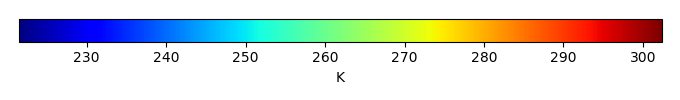MODEL MEANBIAS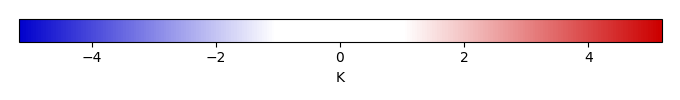BIAS SCORERMSE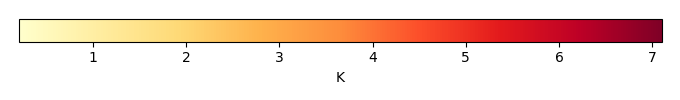RMSE SCOREBENCHMARK INTERANNUAL VARIABILITY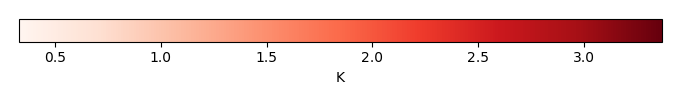MODEL INTERANNUAL VARIABILITYINTERANNUAL VARIABILITY SCOREBENCHMARK MAX MONTHMODEL MAX MONTHDIFFERENCE IN MAX MONTH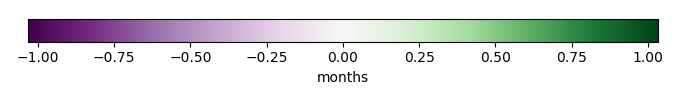SEASONAL CYCLE SCORESPATIAL TAYLOR DIAGRAMMODEL COLORS# Spatially integrated regional mean

MODEL COLORSREGIONAL MEANANNUAL CYCLEMONTHLY ANOMALYANNUAL CYCLE# All Models

BenchmarkCLM4CLM4.5CLM5# Data Information

creation_date: Fri Nov 1 11:52:30 PDT 2013

source_file: This product is generated from monthly CRU TS3.21 Mean Temperature observations

title: derived CRU high resolution (0.5x0.5) Mean Temperature

Approach: I simply read the monthly surface air temperature from original file, and separated the time series by a month and saved them by each month and each year.

Temporal resolution: monthly

General information: This product was derived from CRU v3.21 product.

Spatial resolution: 0.5x0.5 degree